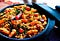# Simple CNN using NumPy Part VI (Putting it all together)

In the previous posts, we covered the following

# The Final Code

`'''Input functionality'''import pandas as pdimport numpy as npdata = pd.read_csv('../input/Kannada-MNIST/train.csv')data = data.sample(frac=1)#print(int(data.shape/2))data_first_half = data.head(30000)data_second_half = data.tail(30000)### get 100 data points ### making sure that the data  is balancedtmp = pd.DataFrame()for label in range(10):    if label==0:        tmp = data_first_half[data_first_half['label']==label].head(600)    else…`

# Simple CNN using NumPy Part V (Back Propagation Through Max pool Layer & Convolutional Filter)

In the previous blog posts , I tried to explain the following

In this post, I will try to cover back propagation through the max pooling and the convolutional layers. We had worked our way through calculating the gradients till the first fully connect layers. Let’s re-visit the architecture once more through the following hand drawn image

# Simple CNN using NumPy Part IV (Back Propagation Through Fully Connected Layers)

In the previous sections , we covered

In the 4th part of this series on CNN, we will try to cover back propagation through the fully connected layers in the network.

Just to recap, our network has the following set of operations in order from start to finish

Let N be the total number of images

• It accepts an input matrix of size (N,1,28,28)
• This is then followed by the first convolutional filter of size (2,1,5,5)
• The convolution operation results in the matrix transforming to the size (N,2,24,24). …

# Recap

In the previous posts, I covered the following topics

In the third part of the series, I will cover three functions that will be used during forward propagation.

# ReLU Function

The ReLU function is a non-linear activation function which filters out negative values.

ReLU function applied in a neural network does not face problems such as vanishing gradients. ReLU function result in non zero gradients for positive values , unlike saturating functions such as sigmoid.

Given that the differential of a ReLU function is a constant, lesser time is need to compute the…

# Simple CNN using NumPy Part II (Convolution Operation)

In the previous post, I gave a brief introduction to convolutional neural networks together with code for converting CSV data of flattened images to their actual shapes. In this post, I will try to explain the following

• Convolution operation
• Why is convolution needed?
• Implementing it using NumPy

# Convolution Operation

In the context of ConvNets, the convolution operation involves calculating the dot products between a fixed matrix and different regions of an image. The fixed matrix is also known as the convolutional filter. The different regions of the image have the same shape as the fixed matrix. …

# Introduction

Convolutional Neural Networks (CNNs) are a class of neural networks that work well with grid-like data, such as images. They extract useful features from images to make the image recognition process more robust. These networks are inspired by the results of experiments conducted by David Hunter Hubel & Torsten Nils Wiesel who observed different neural activity in the cat’s brain in response to different orientations of a straight line.

## First Convolutional Networks

The first convolutional neural network was the Neocognitron, implemented by Dr Kunihiko Fukushima in 1980. This system used a hierarchical structure to learn simple & complex features of an image. …

# Neural Network

Neural Networks are a group of algorithms that consist of computational nodes, that take in an input, perform mathematical computations on it, and return an output. Complex mathematical operations can be performed based on the functions we choose to use on these computational nodes. These functions are also called “activations”.

Neural Networks can be used for the purpose of estimating real values(regression) ,categorical variables (classification) and generating data (generative models).

# The Fashion MNIST data set and data processing

The Fashion MNIST Data set consists of 60,000 28 pixel by 28 pixel black and white images created by Zalando Research . Each image belongs to one of the following…

# Motivation

When working on a regression/classification problem involving data with a large number of features, we are often susceptible to the “Curse of Dimensionality”. As we keep increasing the number of features, we will reach a point where our regressor/classifier fails to perform well on test data.

This is due to the increase in data sparsity brought about by the increase in the number of features in the data. Increased data sparsity enables our models to overfit, thereby failing on future data.

This “Curse” can be avoided by reducing the number of features. This practice is called Dimensionality Reduction.

# Introduction

The Human Activity recognition dataset consists of information collected from embedded accelerometer and gyroscopes while performing tasks like WALKING, WALKING_UPSTAIRS, WALKING_DOWNSTAIRS, SITTING, STANDING, and LAYING. The experiments have been carried out with a group of 30 volunteers within an age bracket of 19–48 years, with video evidence so as to label the observations correctly. The measurements result in a 561 feature vector. The data set for the same can be found at Kaggle.

# The aim of this project

The aim of this project is to effectively predict the observed activities multinomial logistic regression as the predicting algorithm. R will be used in the analysis.

# Multinomial Logistic Regression

Multinomial…“Pot of Spanish paella with shrimp, rice, sausage, and peppers for dinner” by Cel Lisboa on Unsplash

# EDA of the Zomato Dataset using R

Zomato is a restaurant search application which was founded in 2008. It currently operates in 23 countries.

# Libraries

I will be using the `tidyverse` library here for data munging and the `ggplot2` package for data visualization.The data set has been imported using the `read.csv()` function. The data set for this post can be found on Kaggle.

`library(tidyverse)library(ggplot2)library(ggridges)library(tidytext)zomato = read.csv('zomato.csv')zomato %>%  str()`

Where are these restaurants located?

To find out the locations of the restaurant, I have used the world map. Each point on the world map is colored according to the price range they fall in.

`…`